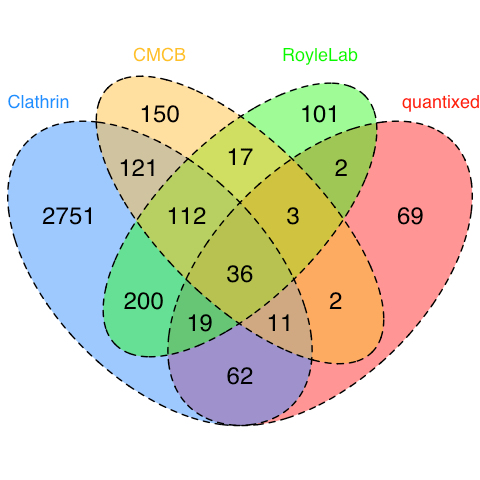Want to share your content on R-bloggers? click here if you have a blog, or here if you don't.

My activity on twitter revolves around four accounts.

I try to segregate what happens on each account, and there’s inevitably some overlap. But what about overlap in followers?

What lucky people are following all four? How many only see the individual accounts?

It’s quite easy to look at this in R.So there are 36 lucky people (or bots!) following all four accounts. I was interested in the followers of the quantixed account since it seemed to me that it attracts people from a slightly different sphere. It looks like about one-third of quantixed followers only follow quantixed, about one-third follow clathrin also and more or less the remainder are “all in” following three accounts or all four. CMCB followers are split about the same. The lab account is a bit different, with close to one-half of the followers also following clathrin.

Extra nerd points:

This is a Venn diagram and not an Euler plot. Venn just shows schematically the intersections and does not attempt to encode information in the area of each part. Euler plots for greater than three groups are hard to generate and to make any sense of what is shown. It is a dataviz problem to look at the proportions or lots of groups. A solution here would be to generate a further four Venn diagrams. On each, display the proportion for one category as a fraction or percentage

How to do it:

Last time, I described how to set up rtweet and make a Twitter app for use in R. You can use this to pull down lists of followers and extract their data. Using the intersect function you can work out the numbers of followers at each intersection. For four accounts, there will be 1 group of four, 4 groups of three, 6 groups of two. The VennDiagram package just needs the total numbers for all four groups and then details of the intersections, i.e. you don’t need to work out the groups minus their intersections – it does this for you.

```library(rtweet)
library(httpuv)
library(VennDiagram)
## whatever name you assigned to your created app
appname <- "whatever_name"
## api key (example below is not a real key)
key <- "blah614h"
## api secret (example below is not a real key)
secret <- "blah614h"
app = appname,
consumer_key = key,
consumer_secret = secret)
clathrin_followers <- get_followers("clathrin", n = "all")
clathrin_followers_names <- lookup_users(clathrin_followers)
quantixed_followers <- get_followers("quantixed", n = "all")
quantixed_followers_names <- lookup_users(quantixed_followers)
cmcb_followers <- get_followers("Warwick_CMCB", n = "all")
cmcb_followers_names <- lookup_users(cmcb_followers)
roylelab_followers <- get_followers("roylelab", n = "all")
roylelab_followers_names <- lookup_users(roylelab_followers)
# a = clathrin
# b = quantixed
# c = cmcb
# d = roylelab
## now work out intersections
anb <- intersect(clathrin_followers_names\$user_id,quantixed_followers_names\$user_id)
anc <- intersect(clathrin_followers_names\$user_id,cmcb_followers_names\$user_id)
and <- intersect(clathrin_followers_names\$user_id,roylelab_followers_names\$user_id)
bnc <- intersect(quantixed_followers_names\$user_id,cmcb_followers_names\$user_id)
bnd <- intersect(quantixed_followers_names\$user_id,roylelab_followers_names\$user_id)
cnd <- intersect(cmcb_followers_names\$user_id,roylelab_followers_names\$user_id)
anbnc <- intersect(anb,cmcb_followers_names\$user_id)
anbnd <- intersect(anb,roylelab_followers_names\$user_id)
ancnd <- intersect(anc,roylelab_followers_names\$user_id)
bncnd <- intersect(bnc,roylelab_followers_names\$user_id)
anbncnd <- intersect(anbnc,roylelab_followers_names\$user_id)
## four-set Venn diagram
area1 = nrow(clathrin_followers_names),
area2 = nrow(quantixed_followers_names),
area3 = nrow(cmcb_followers_names),
area4 = nrow(roylelab_followers_names),
n12 = length(anb),
n13 = length(anc),
n14 = length(and),
n23 = length(bnc),
n24 = length(bnd),
n34 = length(cnd),
n123 = length(anbnc),
n124 = length(anbnd),
n134 = length(ancnd),
n234 = length(bncnd),
n1234 = length(anbncnd),
category = c("Clathrin", "quantixed", "CMCB", "RoyleLab"),
fill = c("dodgerblue1", "red", "goldenrod1", "green"),
lty = "dashed",
cex = 2,
cat.cex = 1.5,
cat.col = c("dodgerblue1", "red", "goldenrod1", "green"),
fontfamily = "Helvetica",
cat.fontfamily = "Helvetica"
);
# write to file
grid.draw(venn.plot);
dev.off()
```

I’ll probably return to rtweet in future and will recycle the title if I do.

Like last time, the post title is from “I’m Not Following You” the final track from the 1997 LP of the same name from Edwyn Collins